## “We are sometimes fascinated to know how Google Translate works to translate a sentence in one language to another language. What could be the basics for mapping one variable length entity to another variable length entity? This makes the path to learn about encoder-decoder networks and how we can make use of some sequence based models such as RNNs and LSTMs to construct them.”

Prerequisites : Knowledge of RNN, LSTM and Neural Networks.

Before we hop onto Encoder-Decoder networks and other technical details. There is an important question as to how do we represent words in a big sentence? Can I simply feed in a sequence of words(consisting of letters) to a model so that it gives a translated sequence as its output? A simple and delicate concept is that we represent words by a vector, a list of numbers or a point in N-dimensional space. Such a representation is usually called embedding. Computers can use this trick to understand the meaning of words in its context which can be subsequently fed into models to perform different tasks. So for translation, we represent the input sequence of words as a sequence of embeddings and feed it into our model to get an output sequence of embeddings representing our translated sequence of words.

Encoder-Decoder Networks:

The Encoder-Decoder LSTM is a recurrent neural network designed to address sequence-to-sequence problems, sometimes called seq2seq.

A machine translation problem and learning to execute programs can also be addressed as a seq2seq problem. Sequence-to-sequence prediction problems are challenging because the number of items in the input and output sequences can vary.

→ What exactly is a seq2seq problem? Sequence prediction often involves forecasting the next value in a real valued sequence or outputting a class label for an input sequence. This is often framed as a sequence of one input time step to one output time step (e.g. one-to-one) or multiple input time steps to one output time step (many-to-one) type sequence prediction problem. There is a more challenging type of sequence prediction problem that takes a sequence as input and requires a sequence prediction as output. These are called sequence-to-sequence prediction problems, or seq2seq for short. One modeling concern that makes these problems challenging is that the length of the input and output sequences may vary. Given that there are multiple input time steps and multiple output time steps, this form of problem is referred to as many-to-many type sequence prediction problem.

So as we proceed, we recall that our translation task is one of the seq2seq tasks where input and output are both variable-length sequences. To handle this type of inputs and outputs, we can design an architecture with two major components. The first component is an encoder : it takes a variable-length sequence as the input and transforms it into a state with a fixed shape. The second component is a decoder : it maps the encoded state of a fixed shape to a variable-length sequence. This is called an encoder-decoder architecture, which is depicted in the figure below: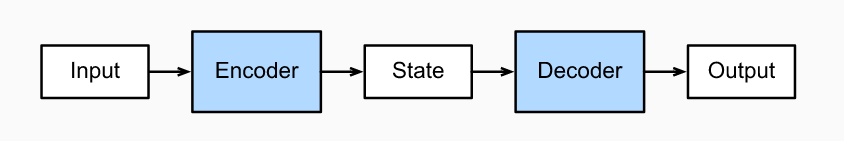For example, let us take machine translation from English to French as an example. Given an input sequence in English: “They”, “are”, “watching”, “.”, this encoder-decoder architecture first encodes the variable-length input into a state, then decodes the state to generate the translated sequence token by token as the output: “Ils”, “regardent”, “.”

At this point of time, one may think and realize how beautifully our encoder-decoder network is able to perform this translation task. But, what any network does is not a magic task, one should note the bottleneck or the core idea behind any algorithm or model.

Why is our network able to do such translation tasks? Is it trying to learn something from a particular language and then correlate it to the other language?

The encoder takes the input sequence and creates a contextual representation called the state (which is also called context) of it and the decoder takes this contextual representation as input and generates output sequence. The core idea lies in the generation of these states where the encoder part of the model tries to learn the representations of one language and once these states are present, the decoder tries to relate the state into the new language we want to translate to.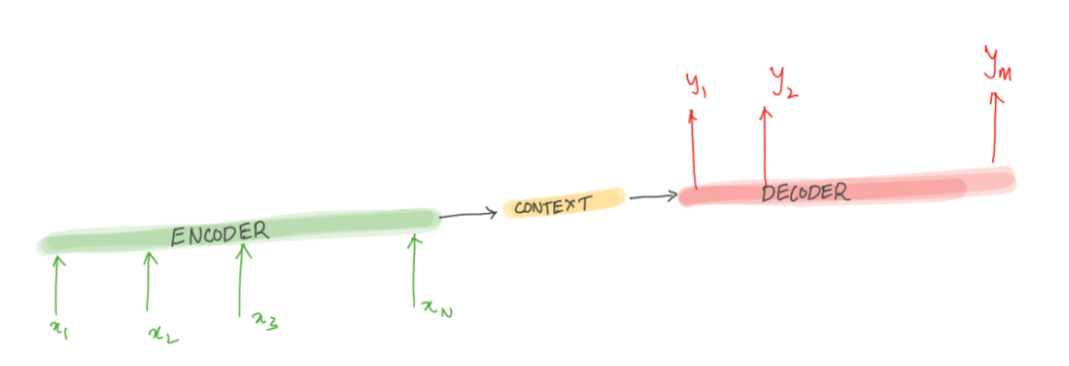While constructing the encoder-decoder network, one may use RNN or LSTM cells in both encoder and decoder parts. For our explanation, the idea is to use one RNN to read the input sequence, one time step at a time, to obtain large fixed-dimensional vector representation, and then to use another RNN to extract the output sequence from that vector. In RNN’s we have a notion of hidden state “ht” which can be seen as a summary of words/tokens it has seen till time step “t” in the sequence chain. One important step before feeding the input sequence of works into the Encoder RNN will be to first convert the sequence into a sequence of embedding for each word.

Analyzing the Encoder Part:

Encoder takes the input sequence and generates a context which is the essence of the input to the decoder. The entire purpose of the encoder is to generate a contextual representation/ context for the input sequence. Using RNN as encoder, the final hidden state of the RNN sequence chain can be used as a proxy for context. This is the most critical concept which forms the basis for encoder-decoder models. We will use the subscripts ‘e’ and ‘d’ for the hidden state of the encoder and decoder. Outputs of the encoder are ignored, as the goal is to generate a final hidden state or context for the decoder.

The figure below shows the use of RNN as encoder: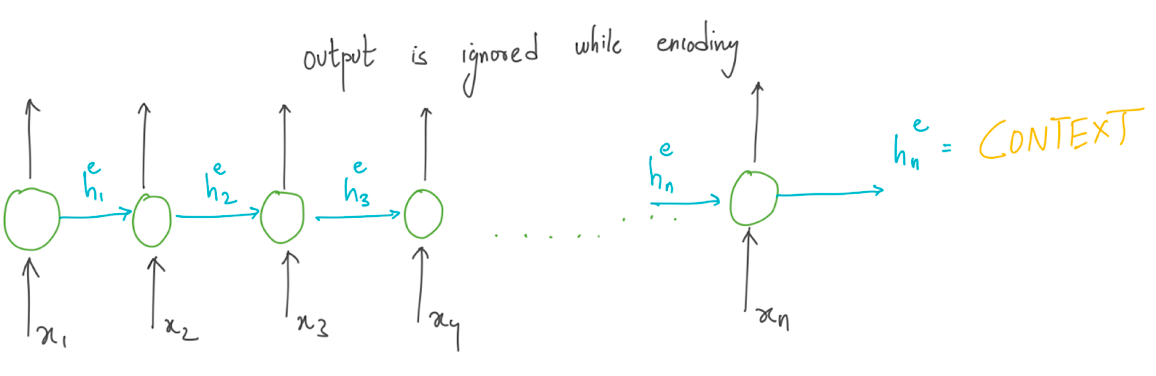Here xi represents the embedding vectors of different words in the input sequence, after processing through n cells of RNN, we get a context vector which will be used as a representative state for the decoder RNN. Till now, the encoder RNN has tried to understand and find some meaning representation out of input sequence of embeddings.

Analyzing the Decoder Part:

Decoder takes the context as input and generates a sequence of output. When we employ RNN as decoder, the context is the final hidden state of the RNN encoder. The first decoder RNN cell takes the context(or the intermediate ‘state’) as its prior hidden state. The decoder then generates the output until the end-of-sequence marker is generated. The EOS (End of State Marker) is used to signify the end token for the sentence.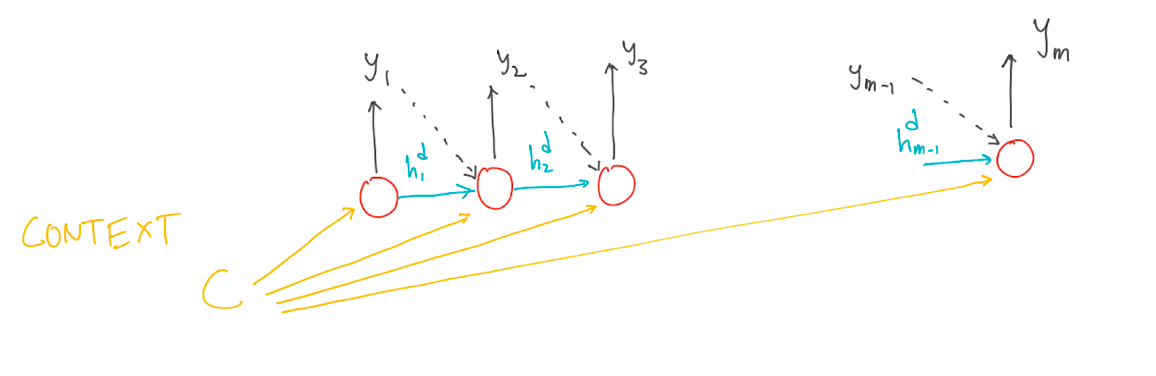Each cell in RNN decoder takes input in an auto regressively manner, i.e, the decoder uses its own estimated output at time ‘t’ as the input for the next time step xt+1. One important drawback is that if the context is made available only for first decoder RNN cells the context wanes as more and more output sequence is generated. To overcome this drawback the context can be made available at each decoding RNN time step. There is a little deviation from vanilla-RNN.

The equations for Decoder RNN can be represented as below: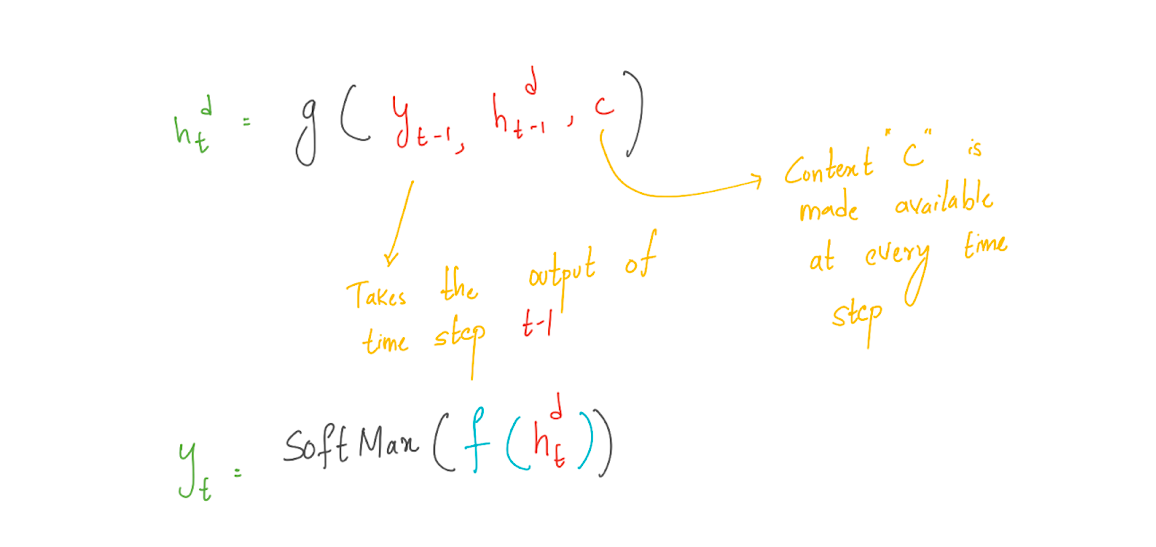Training the model:

The training data consists of sets of input sentences and their respective output sequences. We use cross entropy loss in the decoder. Encoder-decoder architectures are trained end-to-end, just as with the RNN language models. The loss is calculated and then back-propagated to update weights using the gradient descent optimization. The total loss is calculated by averaging the cross-entropy loss per target word.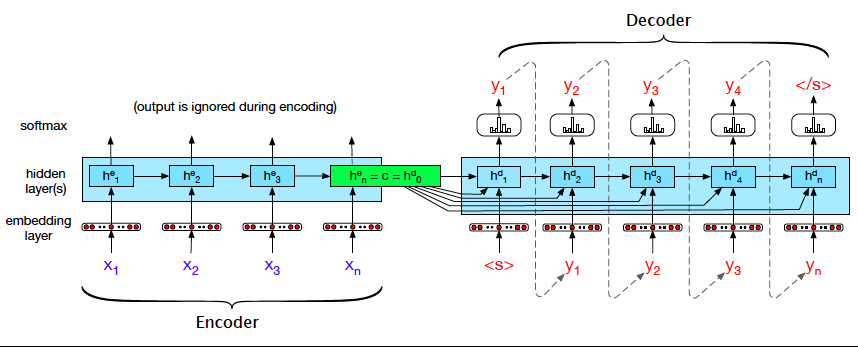If we consider the machine translation task, we train while feeding in the input sequence (x1,x2,….,xn) to the encoder RNN while feeding the target sequence (y1,y2,…..,yn) to the decoder RNN. After training, we try to generate the translated sequence(from the decoder part) by feeding the input sequence to the encoder.

Code:

One can find a good resource on encoder-decoder networks and other variations of it on this link. The code is in Kotlin and can be implemented on the S2 platform.

Summary:

• The encoder-decoder architecture can handle inputs and outputs that are both variable-length sequences, thus is suitable for sequence transduction problems such as machine translation for translation text in one language to another. It can also be used for some time-series forecasting problems where we are required to process the inputs in a sequential manner.

• The encoder takes a variable-length sequence as the input and transforms it into a state with a fixed shape which acts like a representative entity for the decoder.

• The decoder maps the encoded state of a fixed shape to a variable-length sequence which is also our desired sequence.

Limitations:

• Not only do encoder-decoder networks need a comprehensive amount of training data, they also need relevant data. Like many algorithms, encoder-decoder networks are data-specific and data scientists must consider the different categories represented in a data set to get the best results. It is vital to make sure the available data matches the business or research goal; otherwise, valuable time will be wasted on the training and model-building processes.

• Training a simple Encoder-Decoder network may not always work efficiently on a particular task. The RNN or LSTM architecture used in the encoding and decoding parts may not be able to capture long term dependencies in a sequence in both the direction of a sentence while creating its representation. In these cases even complex architectures may be required for modeling which may use better techniques like attention networks.